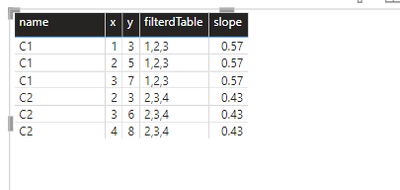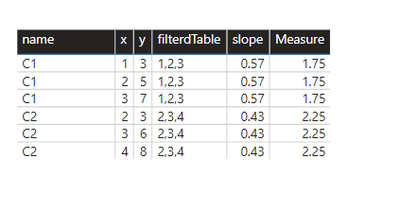cancel
Showing results for
Did you mean:Super User

## LINEST forEach

I am trying to crerate a measure using LINEST that would give me the slope but I have not been successful.

PFA what I have tried.

Following is what I desire

``````| Country | x | y | slope |
|---------|---|---|-------|
| C1      | 1 | 3 | 1.75  |
| C1      | 2 | 5 | 1.75  |
| C1      | 3 | 7 | 1.75  |
| C2      | 2 | 3 | 2.25  |
| C2      | 3 | 6 | 2.25  |
| C2      | 4 | 8 | 2.25  |``````

To the best of my knowledge, I am passing on the correct filtered table to LINESTProud to be a Super User!

New Animated Dashboard: Sales Calendar

2 ACCEPTED SOLUTIONSCommunity Support

Hi @smpa01 ,

``````Measure =
var _a = FILTER(ALL('fact'),'fact'[Country]=MAX('fact'[Country]))
return MAXX(CALCULATETABLE(LINEST('fact'[y],'fact'[x]),_a),[Slope1])``````

Final output:Best Regards,

Jianbo Li

If this post helps, then please consider Accept it as the solution to help the other members find it more quickly.Super User

@smpa01 You could create a measure that returns a text table using TOCSV and parse that.

For example:

``````LINEST Output =
VAR _filteredTable_ = FILTER ( ALL ( 'fact' ), 'fact'[Country] = MAX ( 'fact'[Country] ) )
VAR _linEst_ = LINESTX ( _filteredTable_, 'fact'[y], 'fact'[x] )
VAR _toText = TOCSV ( _linEst_, , "|", FALSE() )
RETURN
_toText``````

Then you can define the following:

``Slope1 = PATHITEM ( [LINEST Output], 1 )``
``ResidualSumOfSquares = PATHITEM ( [LINEST Output], 10 )``
5 REPLIES 5Community Support

Hi @smpa01 ,

``````Measure =
var _a = FILTER(ALL('fact'),'fact'[Country]=MAX('fact'[Country]))
return MAXX(CALCULATETABLE(LINEST('fact'[y],'fact'[x]),_a),[Slope1])``````

Final output:Best Regards,

Jianbo Li

If this post helps, then please consider Accept it as the solution to help the other members find it more quickly.Super User

@v-jianboli-msft  just being curious here. So if I need Total Sum of Square, Residual Sum of Square and R Square, I need to create 3 seperate measures (TSS, RSS and RSquare) and then create subsequent measures for each  ( incorect TSS, RSS, RSquare) immediately created measures like you did above to derive at the correct TSS, RSS, RSquare value for the filter context?

Proud to be a Super User!

New Animated Dashboard: Sales CalendarPower BI Team

You have to create different measures, one for each statistic you need. There is currently no way to create a DAX function that returns a table value to be shared by multiple measures.Super User

@smpa01 You could create a measure that returns a text table using TOCSV and parse that.

For example:

``````LINEST Output =
VAR _filteredTable_ = FILTER ( ALL ( 'fact' ), 'fact'[Country] = MAX ( 'fact'[Country] ) )
VAR _linEst_ = LINESTX ( _filteredTable_, 'fact'[y], 'fact'[x] )
VAR _toText = TOCSV ( _linEst_, , "|", FALSE() )
RETURN
_toText``````

Then you can define the following:

``Slope1 = PATHITEM ( [LINEST Output], 1 )``
``ResidualSumOfSquares = PATHITEM ( [LINEST Output], 10 )``Super User

Thanks @AlexisOlson , applied here

If a linear regreesion is required but only for viz purpose there are tons (I literally mean tons) of javascript library that can do the job at the front-end while developing cust viz and there won't be any DAX dependency.

However, if the linear regression is required as part of the SSAS dataset then DAX is the only option and this is a really nice trick here.

Proud to be a Super User!

New Animated Dashboard: Sales Calendar